Get inspired by the success stories of our students in IIT JAM MS, ISI  MStat, CMI MSc DS.  Learn More

# Shift the Curves | ISI MStat 2019 PSB Problem 1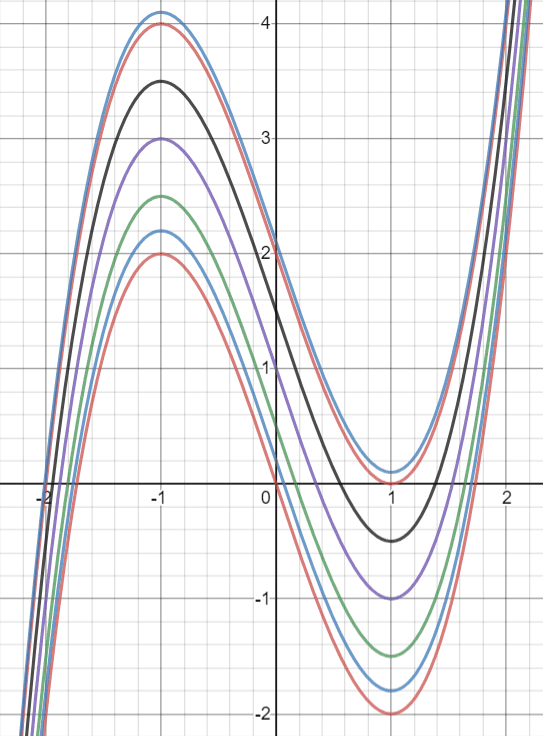This problem is an easy application in calculus using the basic ideas of curve sketching. This is the problem 1 from ISI MStat 2019 PSB.

## Problem- curve sketching

Let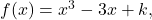where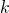is a real number. For what values ofwill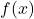have three distinct real roots?

## Solution

Science is all about asking proper questions. So,is the variable here. So, ask the following question,

What role isplaying here? Let's take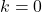and see what happens..

We can easily draw the graph of this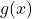. Let's draw it. So,has roots as {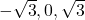}.

Great, so adddingtowill shift the graph upwards right?

Observe that if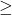|lowest value ofat the bump|, thenwill have less than three roots.

Similarly, observe that if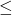- highest value ofat the bump, thenwill have less than three roots.

So, let's find them out. So, what is the mathematical significance of these bumps? They are the local maximum and minimum. How do we find them?, where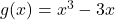.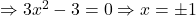.

So, the minimum and the maximum values ofare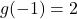and.

Hence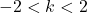.

Let's see what happens at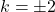.

It is just that point, where the transitition for three roots to two roots occur and then to one root.

Observe that since, it is an odd degree, there will always be a reall root of the curve.

Stay Tuned! Stay Blessed!

## Food for Thought ( Think with Pictures )

•has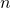distinct roots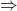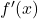has atleast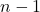distinct roots.
• Is the converse true? i.e.hasdistinct roots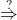has atleastdistinct roots. Can you investigate using the given function?
•hasdistinct roots. Does there exist asuch that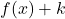has atleastdistinct roots?

Stay Tuned! Stay Blessed!

This problem is an easy application in calculus using the basic ideas of curve sketching. This is the problem 1 from ISI MStat 2019 PSB.

## Problem- curve sketching

Letwhereis a real number. For what values ofwillhave three distinct real roots?

## Solution

Science is all about asking proper questions. So,is the variable here. So, ask the following question,

What role isplaying here? Let's takeand see what happens..

We can easily draw the graph of this. Let's draw it. So,has roots as {}.

Great, so adddingtowill shift the graph upwards right?

Observe that if|lowest value ofat the bump|, thenwill have less than three roots.

Similarly, observe that if- highest value ofat the bump, thenwill have less than three roots.

So, let's find them out. So, what is the mathematical significance of these bumps? They are the local maximum and minimum. How do we find them?, where..

So, the minimum and the maximum values ofareand.

Hence.

Let's see what happens at.

It is just that point, where the transitition for three roots to two roots occur and then to one root.

Observe that since, it is an odd degree, there will always be a reall root of the curve.

Stay Tuned! Stay Blessed!

## Food for Thought ( Think with Pictures )

•hasdistinct rootshas atleastdistinct roots.
• Is the converse true? i.e.hasdistinct rootshas atleastdistinct roots. Can you investigate using the given function?
•hasdistinct roots. Does there exist asuch thathas atleastdistinct roots?

Stay Tuned! Stay Blessed!

This site uses Akismet to reduce spam. Learn how your comment data is processed.

### Knowledge Partner